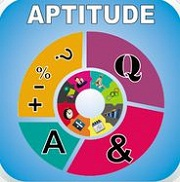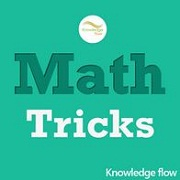# Best Math short cut tricks apps

Here you can get the best Logical Reasoning and Quantitative Aptitude math short cut tricks app for Android Smartphones and tablets. Using this Quantitative Aptitude math short cut tricks apps you can preparation short cut methods and math formula tricks for all competitive exams including IBPS PO and Clerk, SSC, RRB, UPSC, GPSC, LIC, Banking exams, etc. Using this math short cut tricks Android apps you can calculate fastly math problems and save time in competitive exams.

There are several Quantitative Aptitude math short cut tricks Android apps available in play store but below you can get the best Math short cut tricks apps for all competitive exams.

Best Kids Math apps for Windows 8

Top 10 GCSE Maths apps for iPhone

## Top 7 best Math short cut tricks apps / Quantitative Aptitude math short cut tricks / Android apps1) Math Tricks Android app:

This math short cut tricks Android app contains fun math tricks including addition, multiplication, subtraction, division, multiply a two-digit number by 11, power of two, percent, multiply two numbers between 11 & 99, etc. Using this math short cut tricks app for a competitive exam you can easily preparation basic math short cut tricks and formulas.2) Quantitative Aptitude short cut trick Android app:

This Quantitative Aptitude math short cut tricks the Android app contains Age, Calendar, Chain Rule, Simple and Compound Interest, Clock, Decimal Fraction, Boats and Streams, Logarithm, Numbers, Probability, Profit and Loss, Area, etc.

This Quantitative Aptitude math short cut tricks app 2020 also includes 600+ questions for practice and also useful for other competitive exams like CAT, XAT, SAT, GRE, NTSE, GMAT, etc. This Quantitative Aptitude short cut trick Android app is #1 best math short cut tricks apps.3) Quantitative Aptitude math short cut tricks Android app:

This Quantitative Aptitude math short cut tricks Android app 2020 contains the short cut of Profit and loss, Height and Distance, HCM and LCM, Simple and Compound Interest, Averages, Pipes and Cistern, etc.4) Short Tricks of Math Android app:

In this math short cut tricks, the Android app contains short cut tricks for Multiplication, finding Square, Square roots, Cube roots, etc. This basic math short cut trick Android app is the best math short cut tricks apps for all competitive exams 2020.5) Pocket Aptitude Android app:

This Android app contains percentages, Area and Volume, Cubes, Number Series, Mixtures, Frictions, Probability, Ratio, and Proportion, etc. Using this pocket Quantitative Aptitude math short cut tricks you can practice 1800+ questions. These best math short cut tricks apps for Android also useful for CAT, UPSC, MBA, IAS, RRB, GATE, SSC, NTSE, etc.6) Vedic Math Android app in Hindi & English:

This Vedic Math Android app in the Hindi language contains ancient Vedic math short cut tricks including complex numbers, multiplications, divisibility, square and cube roots, decimal fractions, Auxiliary fraction, etc. Using this Vedic math short cut tricks app in the Hindi language you can practice math short questions and short cut formulas. This best Vedic math short cut tricks app in the Hindi Language is best for all competitive exams 2020.